CHAPTER 2: MATRIX COMPUTATIONS AND LINEAR ALGEBRA

Lecture 2.5: Plotting of functions of two variables

Functions on rectangular grids:

Consider a function of two variables: z = f(x,y) in the rectangular domain:

D =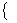(x,y): a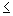xb; cyd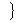Define the discrete interval of the x-axis:

[ a, x1, x2, …, xN, b ]

Define the discrete interval of the y-axis:

[ c, y1, y2, …, yM, d ]

Intersections of vertical lines x = xn and horizontal lines y = ym form a rectangular grid of N-by-M  interior points and (2*N+2*M) boundary points and 4 corner points. Evaluations of the function z = f(x,y) at the grid points defines an (N+2)-by-(M+2) matrix of values: z{n,m} = f(xn,ym).

·         meshgrid(x,y): creates matrices for rectangular grids [X,Y] from vectors [x,y]

x = 0 : 0.5 : 2;  % a row-vector of 5 points for the x-axis

y = -3 : 1 : 3;  % a row-vector of 7 points for the y-axis

[X,Y] = meshgrid(x,y) % create 5-by-7 matrices for grids of X and Y

% meshgrid is equivalent to: X = ones(7,1)*x; Y = y'*ones(1,5);

X        0    0.5000    1.0000    1.5000    2.0000

0    0.5000    1.0000    1.5000    2.0000

0    0.5000    1.0000    1.5000    2.0000

0    0.5000    1.0000    1.5000    2.0000

0    0.5000    1.0000    1.5000    2.0000

0    0.5000    1.0000    1.5000    2.0000

0    0.5000    1.0000    1.5000    2.0000

Y =   -3    -3    -3    -3    -3

-2    -2    -2    -2    -2

-1    -1    -1    -1    -1

0     0     0     0     0

1     1     1     1     1

2     2     2     2     2

3     3     3     3     3

·         mesh(x,y,z): plot a shape of function of two variables on a rectangular grid

x = -2 : 0.1 : 2; y = -2 : 0.1 : 2;

% z = x.*y.*exp(-x.^2 – y.^2) : example of a function of two variables

% direct coding of the function z = f(x,y) does not work because x,y,z are row-vectors

[X,Y] = meshgrid(x,y);

Z = X.*Y.*exp(-X.^2-Y.^2);

% pointwise multiplication works now because X and Y are matrices

mesh(X,Y,Z);

title('a mesh plot of a function of two variables');

xlabel('x'); ylabel('y'); zlabel('z');

·         contour(x,y,z,label): plots contour lines of a function of two variables on a rectangular grid

• level = L, where L is the number of equally spaced contour levels between the minimum and maximum values of z:  zl = zmin+(zmax-zmin)*(l-1)/(L-1)
• level = V, where V is the vector of contour levels given explicitly
• clabel(h): authomatically annotates the contour levels
• clabel(h,'manual'): allows the user to locate the positions of the labels for contour levels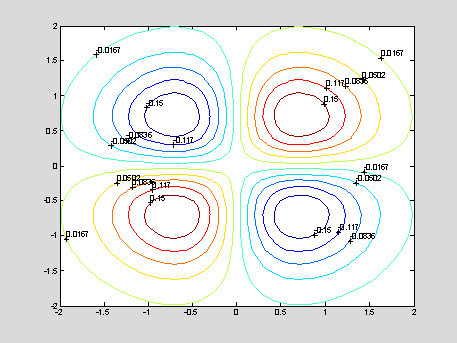h =

·         contour(x,y,z,[0,0]): plots an implicit function of one variable: z = F(x,y) = 0

% y^5 – y^3 = tanh(x) : the implicit function y = y(x) to be plotted

x = -0.3 : 0.01 : 0.3; y = -1 : 0.01 : 1; [X,Y] = meshgrid(x,y);

Z = Y.^5-Y.^3-tanh(X);  contour(x,y,Z,[0,0]); xlabel('x'); ylabel('y');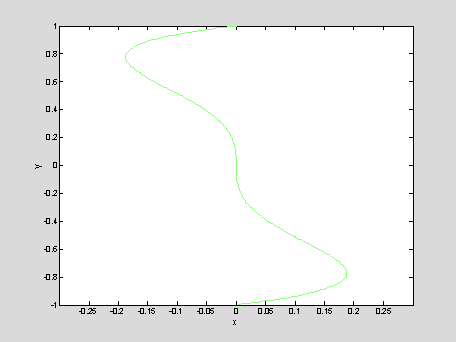·         mesh(x,y,0*y'*x):  plots the rectangular grid without any function on the grid

x = 1 : 0.2 : 3; y = -2 : 0.1 : 2;

mesh(x,y,0*y'*x); view([0,0,1]);

·         surf: creates a colorful image of a two-dimensional surface

x = -2 : 0.1 : 2; y = -2 : 0.1 : 2;

[X,Y] = meshgrid(x,y); Z = X.*Y.*exp(-X.^2-Y.^2);

surf(X,Y,Z); title('a surface defined by a function of two variables');

xlabel('x'); ylabel('y'); zlabel('z');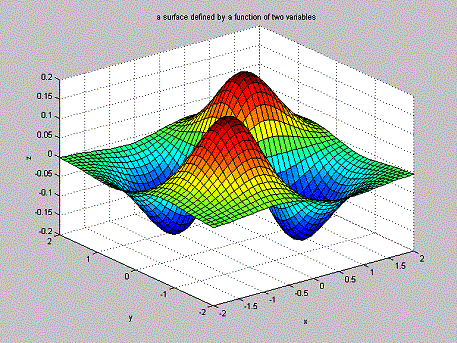·         meshc, surfc: plots contours of the function z = f(x,y) on the (x,y)-plane in addition to the output of functions "mesh" and "surf"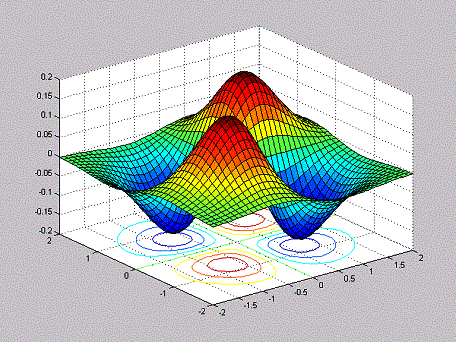·         surfl: creates a two-dimensional surface with lighting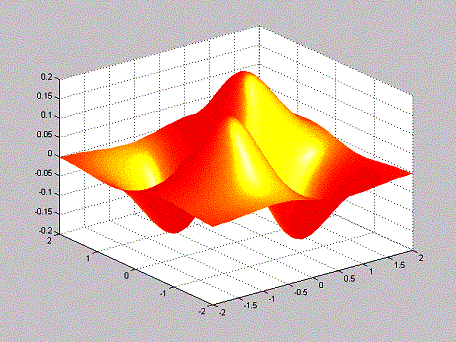·         quiver: plots a two-dimensional vector [u,v] on the plane [x,y], where u = f(x,y); y = g(x,y)

% The system of two differential equations:

% dx/dt = f(x,y) = y; dy/dt = g(x,y) = - sin(x)

% The quiver displays the vector field on the phase plane (x,y)

x = -2*pi:0.25:2*pi; y = -pi:0.25:pi; [X,Y] = meshgrid(x,y);

U = Y; V = -sin(X); quiver(X,Y,U,V,2);

% the last input argument is a scale factor,

% that adjusts the lengths of vectors on the plane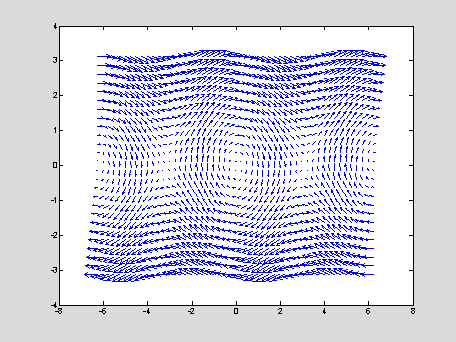More controls of three-dimensional plots:

·         axis([xmin,xmax,ymin,ymax,zmin,zmax]): sets limits of the three-dimensional space

·         xlabel, ylabel, zlabel: labels for x, y, z

·         view([x,y,z]): changes the view angle of the plot, where [x,y,z] are coordinates of a viewer looking at the origin [0,0,0]

·         view([az,el]): changes the view angle of the plot, where az is the azimutal angle in the (x,y) plane, measured from negative y-axis counterclockwise, and el is the elevation angle from the (x,y) plane

• default: view(-37.5,30)

·         colormap: changes definition of colors on the color axis

• default: colormap(hsv), where red is assigned to the lowest and highest values of z, while the intermediate values of z are: red, yellow, green, cyan, blue, magenta, red
• each line of "mesh" is painted by the color determined by the average value of the function at the two adjacent points on the line
• each cell of "surf" is painted by the color determined by the average value of the function at the four corner points of the cell
• other colormaps: hot, cold, jet, gray

x = 1:4; y = 1:8; [X,Y] = meshgrid(x,y); Z = sqrt(X.^2 + Y.^2);

colormap(hsv); surf(Z); xlabel('x'); ylabel('y');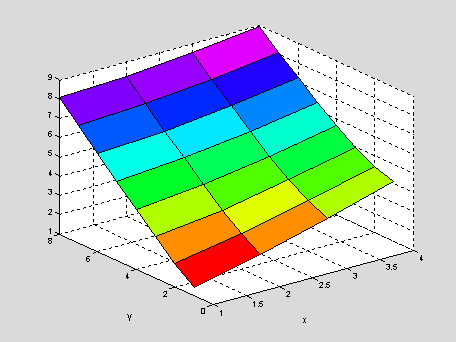·         shading: changes visualiazation of a surface

• faceted: black border lines and quadrilateral tiles
• flat: no border lines
• interp: no border lines and smooth surfaces

·         caxis([zmin,zmax]): specifies the color scheme between the minimal and maximal values of z defined by an user

Remark: it is useful to specify all properties of a figure and keep them with "hold on" before plotting the surface, because plotting operations are time-consuming in space of three dimensions.